•  这是嵌入式课程的一个小作业，用C51单片机实现了0-255内的简易四则运算，暂不支持负数、溢出等特殊情况的处理。 【关键点】  1、计算器用R5、R6、R7三位显示，段码为0，全暗，段码为0xff，全亮。  2、...
【说明】
这是嵌入式课程的一个小作业，用C51单片机，实现了0-255内的简易四则运算，暂不支持负数、溢出等特殊情况的处理。

【关键点】
1、计算器用R5、R6、R7三位显示，段码为0，全暗，段码为0xff，全亮。
2、R3用于保存运算符
3、两数都用B压入栈中，最后取结果时可以分别弹出，并根据操作符计算。
4、程序在两数的第一位默认都输入数字，其他位置皆可复位。
5、一次计算完成后、按任意键复位。

【键盘位置】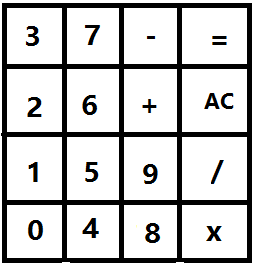代码：
;3位0-255计算器，支持四则运算
;按键有0-9、+、-、*、/、复位、=
;R5,R6,R7分别用于显示三位
;暂不支持负数、溢出处理
ORG		0000H
LJMP	START
WC	EQU	 P0	 ;数码管位控
DC	EQU	 P1  ;数码管段控
KC	EQU	 P2  ;键盘检测

ORG		0030H
START:
MOV 	R5,		#0
MOV 	R6,		#0
MOV 	R7,		#0
N1_B1:
ACALL		KEYDOWN
CJNE		R4,		#0x10,		PRO1		;检测到按键做相应处理
ACALL		DISPLAY
JMP		N1_B1							;否则继续检测
PRO1:
MOV		A,		R4
MOV		R0,		A
MOV		DPTR,	#DCODE
MOVC 		A,		@A+DPTR
MOV		R5,		A
N1_B2:
ACALL		DISPLAY
ACALL		KEYDOWN
CJNE	   	R4,		#0x10,		PRO2		 ;检测按键，有键按下做相应处理
JMP		N1_B2
PRO2:
MOV		A,		R4
ACALL		JUDGE_OPER						 ;检查是否为操作符
CJNE		R3,		#0x04,		CON1		 ;是清除键，直接复位
JMP		START
CON1:
CJNE		R3,		#0x06,		PRO3		 ;是操作符,跳转到PRO3
MOV		A,		R4						 ;是数字
MOV		R1,		A
MOV		DPTR,	#DCODE
MOVC 		A,		@A+DPTR
MOV		R6,		A
MOV		R4,		#0x10
JMP		N1_B3
PRO3:
MOV		B,		R0						 ;将num1的结果保存在B中
PUSH		B
JMP		N2_B1							 ;转去判断第2个数的第1位
N1_B3:
ACALL		DISPLAY							 ;第1个数字第3位
ACALL		KEYDOWN
CJNE	   	R4,		#0x10,		PRO4		 ;检测按键，有键按下做相应处理
JMP		N1_B3
PRO4:
MOV		A,		R4
ACALL		JUDGE_OPER						 ;检查是否为操作符
MOV		A,		R3
CJNE		A,		#0x04,		CON2		 ;是清除键，直接复位
JMP		START
CON2:
CJNE		A,		#0x06,		PRO5		 ;等于则是数字，不等于则是操作符
MOV		A,		R4						 ;是数字
MOV		R2,		A
MOV		DPTR,	#DCODE
MOVC 		A,		@A+DPTR
MOV		R7,		A
MOV		A,		R0						 ;保留第1位数
MOV		B,		#100D
MUL		AB
MOV		R0,		A						 ;将百位保留在R0
MOV		A,		R1						 ;保留第2位数
MOV		B,		#10D
MUL		AB
MOV		R1,		A						 ;将十位保留在R1
MOV		A,		R2						 ;相加
MOV		B,		A						 ;将第1位数先存在B中
PUSH		B
JMP		OPER
PRO5:
MOV		A,		R0
MOV		B,		#10D
MUL		AB
MOV		R0,		A
MOV		A,		R1
MOV		B,		A
PUSH       B
JMP		N2_B1
OPER:
ACALL		DISPLAY
ACALL		KEYDOWN
MOV		A,	   R4
CJNE		A,	   #0x10,		PRO6		 ;有键按下
JMP		OPER
PRO6:
MOV		A,	   R4
ACALL		JUDGE_OPER
MOV		A,	   R3
CJNE		A,	   #0x04,		N2_B1		 ;不等于是操作符,等于是复位键
JMP		START
N2_B1:
ACALL		DISPLAY							 ;第2个数第1位
ACALL		KEYDOWN
MOV		A,		R4
CJNE		A,		#0x10,		PRO7		 ;有键按下
JMP		N2_B1
PRO7:
MOV		A,		R4						 ;保留原有操作符
PUSH		0x03							 ;保存R3中的操作符标记
ACALL		JUDGE_OPER
MOV		A,		R3
POP		0x03
CJNE		A,		#0x04,		CON3		 ;判断是否是复位键
JMP		START
CON3:
MOV		A,		R4
MOV		R0,		A
MOV		DPTR,	#DCODE
MOVC 		A,		@A+DPTR
MOV		R5,		A
MOV		R6,		#0x00		 			 ;清除后两位显示
MOV		R7,		#0X00
N2_B2:
ACALL		DISPLAY
ACALL		KEYDOWN
MOV		A,		R4
CJNE		A,		#0x10,		PRO8		 ;有键按下
JMP		N2_B2

PRO8:
MOV		A,		R4						 ;保留原有操作符
PUSH		0x03							 ;保存R3中的操作符标记
ACALL		JUDGE_OPER
MOV		A,		R3
POP		0x03
CJNE		A,		#0x04,		CON4		 ;不是清除键继续判断
JMP		START
CON4:
CJNE		A,		#0x06,		PRO9		 ;不相等说明是操作符，相等说明是数字
MOV		A,		R4
MOV		R1,		A
MOV		DPTR,	#DCODE
MOVC 		A,		@A+DPTR
MOV		R6,		A
JMP		N2_B3
PRO9:
CJNE		A,		#0x05,		MIDNODE		 ;不是等于号，出错返回
MOV		A,		R0
MOV		B,		A
PUSH		B
JMP		EDIS
N2_B3:
ACALL		DISPLAY
ACALL		KEYDOWN
MOV		A,		R4
CJNE		A,		#0x10,		PRO10
JMP		N2_B3
PRO10:
MOV		A,		R4						 ;保留原有操作符
PUSH		0x03							 ;保存R3中的操作符标记
ACALL		JUDGE_OPER
MOV		A,		R3
POP		0x03
CJNE		A,		#0x04,		CON5		 ;不是清除键继续判断
JMP		START
CON5:
CJNE		A,		#0x06,		PRO11		 ;不相等说明是操作符
MOV		A,		R4
MOV		R2,		A
MOV		DPTR,	#DCODE
MOVC 		A,		@A+DPTR
MOV		R7,		A
MOV		A,		R0
MOV		B,		#100D
MUL		AB
MOV		R0,		A
MOV		A,		R1
MOV		B,		#10D
MUL		AB
MOV		R1,		A
MOV		A,		R2
MOV		B,		A
PUSH		B
JMP		AK
MIDNODE:
JMP		START
PRO11:
CJNE		A,		#0x05,		MIDNODE		   ;通过中继节点返回
MOV		B,		#10D
MOV		A,		R0
MUL		AB
MOV		B,		A
PUSH		B
JMP		EDIS
AK:
ACALL		DISPLAY
ACALL		KEYDOWN
MOV		A,		R4
CJNE		A,		#0x10,		EDIS
JMP		AK
EDIS:
POP	    B
MOV		A,		B
POP		B
MOV		R0,		A		   ;交换AB
MOV		A,		B
MOV		B,		R0
MOV		R1,		A
MOV		A,		R3
CJNE		A,		#0x00,	   NXT1		    ;通过R3的值，判断运算符
MOV		A,		R1						;并进行相应运算
JMP		TS
NXT1:
CJNE		A,	    #0x01,	   NXT2
MOV		A,		R1
SUBB		A,		B
JMP		TS
NXT2:
CJNE		A,		#0x02,	   NXT3
MOV		A,		R1
MUL		AB
JMP		TS
NXT3:
CJNE		A,	   	#0x03,     MIDNODE
MOV		A,	   	R1
DIV		AB
TS:											   ;转换显示
MOV		R0,		A
MOV		B,		#100D
DIV		AB
MOV		R1,		A
MOV		DPTR,	#DCODE
MOVC 		A,		@A+DPTR
MOV		R5,		A
MOV		A,		B
MOV		B,		#10D
DIV		AB
MOV		DPTR,	#DCODE
MOVC		A,		@A+DPTR
MOV		R6,		A
MOV		A,		B
MOV		DPTR,	#DCODE
MOVC		A,		@A+DPTR
MOV		R7,		A
RESTART:
ACALL		DISPLAY
ACALL		KEYDOWN
MOV		A,		R4
CJNE		A,		#0x10,	MIDNODE
JMP		RESTART

;按键检测程序，若有键按下，返回0-15，无键按下返回16
KEYDOWN:
MOV		R4,		#0x10
MOV 	KC,		#0x0F
MOV 	A,		KC
CJNE 	A,		#0X0F,		PRO 	;检测列
RET
PRO:
ACALL 	DELAY				 		;延时消抖
MOV 	A,		KC
CJNE 	A,		#0X0F,		COL		;确认有键按下
RET
COL:
COL1:
MOV 	A,		KC
CJNE 	A,		#0X07,		COL2	;判断哪一列
MOV 	R4,		#0H
JMP 	ROW
COL2:
CJNE 	A,		#0X0B,		COL3
MOV 	R4,		#4H
JMP 	ROW
COL3:
CJNE 	A,		#0X0D,		COL4
MOV 	R4,		#8H
JMP 	ROW
COL4:
CJNE 	A,		#0X0E,		BACK        ;没有列被按下，直接返回
MOV 	R4,		#0CH
ROW:
MOV 	KC,		#0XF0            		;行检测
MOV 	A,		KC
ROW1:
CJNE 	A,		#0X70,		ROW2
MOV 	A,		R4
MOV	 	R4,		A
JMP 	BACK
ROW2:
CJNE 	A,		#0XB0,		ROW3
MOV 	A,		R4
MOV 	R4,		A
JMP 	BACK
ROW3:
CJNE 	A,		#0XD0,		ROW4
MOV 	A,		R4
MOV 	R4,		A
JMP 	BACK
ROW4:
BACK:
MOV		A,		R4
CJNE	A,		#0x10,	DY
RET
DY:
ACALL	DELAY
ACALL 	DELAY
ACALL	DELAY
ACALL	DELAY
RET

;数码管显示3位数字，分别存在R5，R6，R7
DISPLAY:
PUSH	0x00
PUSH	0x01
MOV 	R0,		#0
;输出位码
MOV 	DPTR,	#WCODE
MOV 	A,		R0
MOVC 	A,		@A+DPTR
MOV 	WC,		A
;输出段码
MOV     A,		R5
MOV		DC,		A
MOV		R1,		#0x0f
LP1:
;延长单位显示时间
DJNZ 	R1,		LP1
MOV		DC,		#0
INC 	R0

;输出位码
MOV 	DPTR,	#WCODE
MOV 	A,		R0
MOVC 	A,		@A+DPTR
MOV 	WC,		A
;输出段码
MOV     A,		R6
MOV		DC,		A
MOV		R1,		#0x0f
LP2:
;延长单位显示时间
DJNZ 	R1,		LP2
MOV		DC,		#0
INC 	R0

;输出位码
MOV 	DPTR,	#WCODE
MOV 	A,		R0
MOVC 	A,		@A+DPTR
MOV 	WC,		A
;输出段码
MOV     A,		R7
MOV		DC,		A
MOV		R1,		#0x0f
LP3:
;延长单位显示时间
DJNZ 	R1,		LP3
MOV		DC,		#0
POP		0x01
POP		0x00
RET

;判断A中是什么操作符，+ — * / reset =分别对应R3中的值为0-5，
;若不是操作符，则R3中的值被设置为6
JUDGE_OPER:
MOV		R3,		#6
CJNE		A,		#0x0a,		SB			 ;不是加号，跳到减号
MOV		R3,		#0						 ;0代表加法
RET
SB:
CJNE		A,		#0x0b,		ML			 ;不是减号，跳到乘号
MOV		R3,		#1						 ;1代表减法
RET
ML:
CJNE       A,		#0x0c,		DV			 ;不是乘号，跳到除号
MOV		R3,		#2						 ;2代表乘法
RET
DV:
CJNE		A,		#0x0d,		CL			 ;不是除号，跳到清除键
MOV		R3,		#3						 ;3代表除法
RET
CL:
CJNE		A,		#0x0e,		EU			 ;不是清除键，跳到等号
MOV		R3,		#4						 ;4代表清除键
RET
EU:
CJNE		A,		#0x0f,		FN			 ;不是等号，返回
MOV		R3,		#5						 ;5代表等号
FN:
RET

;延时程序
DELAY:
PUSH 	0x00			;保护现场,R0,R1
PUSH 	0x01
MOV 	R0,		#96H
LP4:
MOV 	R1,		#82H
LP5:
DJNZ 	R1,		LP5
DJNZ 	R0,		LP4
POP 	0x01			;还原现场
POP 	0x00
RET

WCODE: DB  0xfe,0xfd,0xfb,0xf7,0xef,0xdf,0xbf,0x7f		   			;位码
DCODE: DB  0x3f,0x06,0x5b,0x4f,0x66,0x6d,0x7d,0x07,0x7f,0x6f     	;段码
NUM1:  DB	0xff,0xff,0xff											;操作数1
NUM2:  DB  0xff,0xff,0xff											;操作数2
END
展开全文汇编 单片机
• 中国石油大学(北京) 课程设计报告 课程名: 硬件综合实践 姓 名 许恺 学 号 2014011329 班 级 计算机14-1班 设计时间 2018年1月5日 ...在51单片机上编写烧录十进制...


中国石油大学(北京)

课程设计报告

课程名:  硬件综合实践

姓    名   许恺

学    号   2014011329

班    级   计算机14-1班

设计时间   2018年1月5日

一、 设计内容
在51单片机上编写烧录十进制四则运算计算机程序，使之具有计算、纠错、显示、括号运算（甚至浮点数运算）的功能。

二、 设计思路
根据实习设计要求，经过分析可得整个实验可以分为两个部分，软件和硬件，要明白硬件结合软件的方式以及LCD液晶屏的工作方式和51芯片的编码方式以及按钮的电路连接方式；软件要明白多位数四则运算的代码算法和C的语法。
设计思路可以分为三部分。第一，键盘按钮的扫描；第二，芯片内部的运算；第三，LCD液晶的显示；其中第二部分最为复杂重要。

三、 设计解释
3.1、设计环境介绍
51单片机，keil uv3开发软件，烧写软件PZISP自动下载软件.exe，SMC1602A LCM液晶显示屏。
3．2、程序解释（可含硬件部分）
连线：JP8连JP4  JP9连JP5  J8断开  J2断开  JP165断开  把1602液晶插入

代码：
/*
计算器硬件综合实验
*/
#include
<reg51.h>         //此文件中定义了51的一些特殊功能寄存器
#include
<string.h>
#define uchar
unsigned char
sbit EN=P2^7;  //LCD的使能引脚
sbit RS=P2^6;  //LCD数据命令选择端
sbit RW=P2^5;  //LCD的读写选择端
sbit K1=P3^0;      //*号
sbit K2=P3^1;      // /号
sbit K3=P3^2;   //（号
sbit K4=P3^3;      // ）号
sbit K5=P3^4;      // =号
//这里连接了线并且将每位都对应上
code uchar
KEY_CODE[]={ 0xed,0xdd,0xbd,0x7d,0xeb,0xdb,0xbb,0x7b,0xe7,0xd7,0xb7,0x77,    //3X4矩阵键盘键值表
0x7f,0xbf,0xdf,0xef,0xf7};
//2*4键盘键值表
//定义字符键值表，均为字符对应的ASCII码的十六进制
code uchar
CHAR_TABLE[]={0x30,0x31,0x32,0x33,//这四个会在液晶显示器中显示0 1 2 3
0x34,0x35,0x36,0x37,//这四个会显示4 5 6 7
0x38,0x39,0x2b,0x2d,//这个四个会显示8
9 + -
0x2a,0x2f,0x28,0x29,//这四个会显示 *
/ （ ）
0x3d};         //这个会显示 =

//定义优先级矩阵，分为四种情况，当前的比上一个
0优先级高、2优先级低、1左括号遇到右括号、3发生错误
code uchar yxj={
//  + - * / (  ) =
2,2,0,0,0,2,2, // +
2,2,0,0,0,2,2, // -
2,2,2,2,0,2,2, // *
2,2,2,2,0,2,2, // /
0,0,0,0,0,1,3, // (
2,2,2,2,3,2,2, // )
};
uchar str;//定义一个队列，用来放整个运算式
void scan(uchar
*var);//从矩阵键盘中获取值
void print(int
i,int j,uchar *outStr);//打印字符串
void delay5MS();//短延时函数
void delay100MS();//较长延时函数
void writeCMD(uchar
com);//写命令子程序
void showOneChar(uchar
dat);//写数据子程序
void init();//初始化子程序，初始化液晶显示屏
void clear();//清除显示屏上的显示
int calculate(uchar
*arr);//计算函数，输入运算式，返回最终得数
int Precede(char
a,char b);//优先级比较函数，输入两个符号，返回0123哪种情况
int Operate(int
num1,uchar theta,int num2);//计算函数，输入两个数一个符号，返回运算结果

void main()
{
uchar new=0xff; //new是用来放当前扫描到的字符
int i=0,j=0,flag=0;//flag用来确认括号是否匹配，遇到左括号++，右括号--
int finvalue;//存放最后的得数
uchar text;
uchar text1; //在最后用作字符串逆序
delay100MS();
//这是必要的，根据1602显示屏的说明书，使用前必须进行初始化
init();
//使用前清屏
clear();
while(1)
{
flag=0;
while(1)
{
scan(&new);
clear();
//加入出错判断机制，整个出错判断机制主要是选出所有正确的，不正确的根本不会被加到运算式的字符串中，在显示屏上就是按了不显示。如果有浮点数的话就加上小数点的情况
if(new=='='&&flag==0)  //如果new输入=且括号没有问题
{
str[i++]=new;
str[i]='\0';
}
else
if(new<'0'||new>'9')   //若现在输入是符号
{
if(i==0&&new=='(')        //如果第一个要输入（
{
flag++;
str[i++]=new;
}
else
if(i!=0&&(str[i-1]=='+'||str[i-1]=='-'||str[i-1]=='*'||str[i-1]=='/'||str[i-1]=='(')&&new=='(')      //前一个是符号时对现在的进行限制
{
flag++;
str[i++]=new;
}
else
if(i!=0&&str[i-1]==')') //前一个符号是）的时候
{
if(new!='('&&new!=')')
//现在的不是（）的时候可以加进去
str[i++]=new;
else
if(new==')'&&flag>0)
//需要）的时候）后面可以是）
{
flag--;
str[i++]=new;
}
}
if(i!=0&&(str[i-1]>='0'&&str[i-1]<='9')&&new!='(')//前一个是数字时对现在的输入限制
{
if(new==')'&&flag>0)
//如果需要）数字后面可以加）
{
flag--;
str[i++]=new;
}
if(new!=')') //否则数字后面加什么都行
str[i++]=new;
}
}
else     //若现在输入是数字
{
if(i==0) //第一位可以是数字
str[i++]=new;
else
{
if(str[i-1]!=')')
//只要前一位不是），就可以接数字
{
if(str[i-1]!='/'||new!='0')
//排除/后面接0的可能
str[i++]=new;
}
}
}
//出错判断机制结束，下面把内容显示到显示屏上
print(0,0,&str);
if(new=='='&&flag==0)
//如果现在的符号是=且括号对应正确就直接跳出去进行下一次计算，不过前面出错判断机制规定如果括号不对根本就输不出来=，所以不会括号不对应
{
break;
}
}
finvalue=calculate(&str);
//根据运算式计算得出最终得数
if(finvalue<0)      //判断得数正负，负就在最后输出的式子第一位置-
{
finvalue=-finvalue;
text1='-';
}
else text1=' '; //否则就第一位置空格
for(i=0;finvalue%10!=0;i++) //把整型的结果变成字符串型，不过出来后是逆序的
{
text[i]=finvalue%10+0x30;
finvalue/=10;
}
for(j=1;j<i+1;j++) //把逆序的结果反转一下
{
text1[j]=text[i-j];
}
print(1,0,&text1); //把结果显示在屏幕的第二行
i=0;
}
}
/*********************短延时函数*************************/
void delay5MS()
{
int n=3000;
while(n--);
}
/*****************定义长点的延时程序**********************/
void delay100MS()
{
int n=10000;
while(n--);
}

/*下图是LCD显示屏的16位引脚的意思，主要使用的就是RS,RW,E三个来控制LCD的操作，D0-D7来进行数据传输，根据下下图说明51单片机默认LCD显示屏的数据传输口与P0口对应

/*******************写命令子程序**************************/

void writeCMD(uchar
com)
{
P0=com;
//com为输入的命令码。通过P0送给LCD
RS=0;      //RS=0 写命令
RW=0;
delay5MS();
EN=1;      //LCD的使能端E置高电平
delay5MS();
EN=0;       //LCD的使能端E置低电平
}

/*******************写数据子程序**************************/

void
showOneChar(uchar dat)
{
P0=dat;         //写入数据
RS=1;       //RS=1写命令
RW=0;
EN=1;
delay5MS();
EN=0;
}
/*******************初始化函数**************************/

/*其实1602说明书给的很明确了，显示屏的初始化必须这样做*/
void init()
{
EN=0;
writeCMD(0x38);//设置显示模式
writeCMD(0x0e);//光标打开，不闪烁
writeCMD(0x06);//写入一个字符后指针地址+1，写一个字符时整屏不移动
writeCMD(0x01);//清屏显示，数据指针清0，所以显示清0
writeCMD(0x80);//设置字符显示的首地址
}
/*********************清屏子程序**********************/

void clear()
{
EN=0;
writeCMD(0x01);
}
/**********从键盘获取值得函数类似于C语言的scanf()函数**************/
/*这是我设计思路的第一部分，键盘扫描，分为两部分，3*4键盘部分，和2*4部分，两部分用的方式不一样，然后通过已有的字符对照表把参数var置成扫描到的字符。如果加上浮点数的话就增加扫描到小数点时的情况*/
void scan(uchar
*var)
{
uchar temp,num;
int i=1;
temp=i;
while(1){
//3*4键盘扫描，因为有12个键只有8个接口，所以通过3行4列来定为按键，故分为行检测和列检测，为什么要分开呢?是因为下图中，如果直接行列同时检检测的话是不可能检测的，两个口必须一高一低才会有电流通过，同时置0或f是不会产生电流的

P1=0x0f;//置行为高电平，列为低电平。这样用于检测行值。低电平有效
if(P1!=0x0f)
{
delay100MS(); //延时，软件消除抖动。
temp=P1; //保存行值
P1=0xf0; //置行为低电平，列为高电平，获取列
if(P1!=0xf0)
{
num=temp|P1; //获取了按键位置
//P2=1;
for(i=0;i<12;i++)
if(num==KEY_CODE[i])
{
if(i==10)
*var='+';//获取等号的值
else
if(i==11)
*var='-';//获取加号的值
else
*var=i+0x30;//获取数值的字符
}
break;         //跳出循环，为了只获取一个值
}
}
//检测2*4键盘的值，接口足够，所以直接对应，按下为低电平则扫描到

if(K1==0)
{
delay100MS();
*var='*';
break;
}
if(K2==0)
{
delay100MS();
*var='/';
break;
}
if(K3==0)
{
delay100MS();
*var='(';
break;
}
if(K4==0)
{
delay100MS();
*var=')';
break;
}
if(K5==0)
{
delay100MS();
*var='=';
break;
}
}
}
/******************显示函数***************************/
/*i为要输出的行，0为第一行，1为第二行，j为输出的列，arr为输出的字符串通过调用传输数据函数一个一个输出到显示屏上*/
void print(int
i,int j,uchar *arr)
{
int t;
int len=strlen(arr);
int location;
if(i==0)
{
writeCMD(0x80+j);
}
else
{
writeCMD(0xC0+j);
}

if(len==0) //如果队列为空就清屏
{
clear();
return;
}
else
{
for(t=0;t<len;t++)
{
switch(arr[t])
{
case
'0':location=0;break;
case
'1':location=1;break;
case
'2':location=2;break;
case
'3':location=3;break;
case
'4':location=4;break;
case
'5':location=5;break;
case
'6':location=6;break;
case
'7':location=7;break;
case
'8':location=8;break;
case
'9':location=9;break;
case
'+':location=10;break;
case
'-':location=11;break;
case
'*':location=12;break;
case
'/':location=13;break;
case
'(':location=14;break;
case
')':location=15;break;
case
'=':location=16;break;
}

showOneChar(CHAR_TABLE[location]);
}
}

}
/*********************计算字符串输入的运算式的结果**********************/
int calculate(uchar
*arr)
{
int num={0};              //num为数据栈，初值为0
uchar sign;               //sign为符号栈
int i=0,j=0,k=0;    //j是数据栈的栈顶指针，指向栈顶的下一个，k是符号栈的栈顶的下一个
uchar c;    //用来存放当前的字符
int flagnum=0;   //指当前遇到数字的位数
uchar theta;        //运算时的运算符号
int num1,num2,num0;   //运算时的运算数和得数
c=arr[i];
i++;
while(c!='\0')
{

//如果要加上浮点数就增加一种情况就是遇到‘.’的时候改变一下迭代方式
if(c>='0'&&c<='9') //如果当前遇到的是数字，先把字符变成数字，
{
c=c-0x30;
if(flagnum==0)
{
num[j]=c;j++;
//如果这是第一位就直接入栈
flagnum++;
}
else
{
num[j-1]=num[j-1]*10+c;     //不是第一位就拿出来上一个乘十加上再压进去
flagnum++;
}
c=arr[i];i++;
}
else   //如果来的不是数字
{
if(k==0) //如果符号栈里没东西直接入栈
{
sign=c;
k++;
c=arr[i];i++;
flagnum=0;
}
else //符号栈里有东西就得比较一下了，分三种情况
switch(Precede(sign[k-1],c))
{
case
0:     sign[k]=c;k++;c=arr[i];i++;  //当前符号优先级更高则入符号栈
flagnum=0;                        //数字位数清零
break;
case
1:   k--;sign[k]='\0';c=arr[i];i++;break;
//当两个括号相遇时直接出栈
case
2:   theta=sign[k-1];k--;        //当当前的符号优先级小于等于上一个符号时，将栈内的数字和符号取出进行运算
num2= num[j-1];j--;
num1=num[j-1];j--;
num0=Operate(num1,theta,num2);
num[j]=num0;j++; //运算结果入栈
break;
default:  break;
}
}
}
i=0;
while(arr[i]!='\0') //把运算式字符串置为空
{
arr[i]='\0';
i++;
}
return num[j-1]; //返回整型的结果
}
//符号优先级比较，输入a，b两个符号，根据优先级表返回他的优先级对比情况，分为0123
int Precede(char
a,char b)
{
char i,j;
switch(a)
{
case '+':i=0;break;
case '-':i=1;break;
case '*':i=2;break;
case '/':i=3;break;
case '(':i=4;break;
case ')':i=5;break;
case '=':i=6;break;
}
switch(b)
{
case '+':j=0;break;
case '-':j=1;break;
case '*':j=2;break;
case '/':j=3;break;
case '(':j=4;break;
case ')':j=5;break;
case '=':j=6;break;
}
return yxj[i][j];
}
//栈中数的运算，输入两个数一个符号返回一个得数
int Operate(int
num1,uchar theta,int num2)
{
int num3;
switch(theta)
{
case
'+':num3=num1+num2;break;
case
'-':num3=num1-num2;break;
case
'*':num3=num1*num2;break;
case
'/':num3=num1/num2;break;
}
return num3;
}
四、 设计体会与建议
这次的硬件综合实习让我在两周的时间内快速学习了单片机并且进行了实验，虽然之前参加机器人大赛已经对51单片机的烧录和写代码已经有了一定的了解，但是这次的学习让我对它的了解更加深入，前面的几个小实验也是层层递进为最后的实验做准备，四则运算算法一直是我不太会的算法，通过这次实验我学会了，相信在以后也能自如的写出来。本次设计学习了很多东西，在网上查了好多资料，在短时间内对我自身有一个明显的提高，同时也增加了一些未来的可能性，很感谢我的队友徐志远同学，我们两个缺一不可，在这次实验中帮了我很多，也感谢老师对我的鼓励。
徐朝农老师的教学精神值得所有老师学习，既没有轻松水过，也没有过于严格苛刻，高标准+人性化的评判，真正让同学们学到东西。要说建议也没有什么，就是下一届和后面的学弟学妹人数会越来越多，可以申请多一点教具和教室，难度还可以加大。

参考：https://wenku.baidu.com/view/92c3647d650e52ea551898e2.html?qq-pf-to=pcqq.c2c
转载于:https://www.cnblogs.com/xukaiae86/p/8252114.html
展开全文• 基于单片机的多功能计算器设计 extern bit b_WorkMode; //0--计算器模式； //1--计时模式。 //定义在KeyProcess.c中。 unsigned char uc_ModeChange=0; extern bit b_LCDClean; extern ...程序
• 利用矩阵键盘实现一个简易的计算器。 为了简化问题，我们假设只支持小于100的非负整数之间的加、减、乘的运算，并且支持连续运算（结果的数值可以再进行运算）。 本程序中C为加号，D为减号，E为乘号，F为等于号。 ...
利用矩阵键盘实现一个简易的计算器。
为了简化问题，我们假设只支持小于100的非负整数之间的加、减、乘的运算，并且支持连续运算（结果的数值可以再进行运算）。
本程序中C为加号，D为减号，E为乘号，F为等于号。
代码中有详细的注释。
/*   注：本程序 C 为+， D 为- E为* F 为=号，支持非负整数连续运算。
输入的数值小于100，运算结果不超过1000.
by Tach

------------------------------------------------*/
#include<reg52.h>

#define DataPort P0 //定义数据端口 程序中遇到DataPort 则用P0 替换
#define KeyPort  P3

sbit DUAN=P2^6;//定义锁存使能端口 段锁存
sbit WEI=P2^7;//                 位锁存

unsigned char code dofly_DuanMa[]={0x3f,0x06,0x5b,0x4f,0x66,0x6d,0x7d,0x07,0x7f,0x6f,
0x77,0x7c,0x39,0x5e,0x79,0x71,0x40};// 显示段码值0~F和-号
unsigned char code dofly_WeiMa[]={0xfe,0xfd,0xfb,0xf7,0xef,0xdf,0xbf,0x7f};//分别对应相应的数码管点亮,即位码
unsigned char TempData; //存储显示值的全局变量

void DelayUs2x(unsigned char t);//us级延时函数声明
void DelayMs(unsigned char t); //ms级延时
void Display(unsigned char FirstBit,unsigned char Num);//数码管显示函数
unsigned char KeyScan(void);//键盘扫描
unsigned char KeyPro(void);
void Init_Timer0(void);//定时器初始化
/*------------------------------------------------
主函数
------------------------------------------------*/
void main (void)
{
unsigned char num,tempp=0;
int datanum;
int i=0,j,flag,ans,ans_clear,t;
unsigned char temp;
Init_Timer0();

while (1)         //主循环
{
num=KeyPro();
if(num!=0xff)
{

if(num>=0 && num<=9)
{
if(ans_clear)
{
ans_clear=0;
for(j=0;j<8;j++)//清屏
TempData[j]=0;
}
tempp=tempp*10+num;
if(tempp/10>0)
TempData=dofly_DuanMa[tempp/10];
TempData=dofly_DuanMa[tempp%10];
datanum[i]=tempp;

}
else if(num==15)
{
i=0;
tempp=0;
{
ans=datanum+datanum;
t=ans;
if(ans/100>0)
{
TempData=dofly_DuanMa[ans/100];
ans=ans%100;
}
if(ans/10>0 || (TempData!=0 && ans/10==0))
TempData=dofly_DuanMa[ans/10];
TempData=dofly_DuanMa[ans%10];
}
else if(sym_sub==1)
{
ans=datanum-datanum;
t=ans;
if(ans<0)
{
flag=1;
ans=-ans;
}
else
flag=0;

if(flag)
TempData=dofly_DuanMa; //负号

if(ans/100>0)
{
TempData=dofly_DuanMa[ans/100];
ans=ans%100;
}
if(ans/10>0)
TempData=dofly_DuanMa[ans/10];
TempData=dofly_DuanMa[ans%10];

}
else if(sym_mul==1)
{
ans=datanum*datanum;
t=ans;
if(ans/100>0)
{
TempData=dofly_DuanMa[ans/100];
ans=ans%100;
}
if(ans/10>0 || (TempData!=0 && ans/10==0))
TempData=dofly_DuanMa[ans/10];
TempData=dofly_DuanMa[ans%10];
}

sym_sub=0;
sym_mul=0;
ans_clear=1;

datanum=ans;

}
else if(num>=12 && num<=14)
{
i++;
if(num==12)
else if(num==13)
sym_sub=1;
else if(num==14)
sym_mul=1;

tempp=0;

for(j=0;j<8;j++)//清屏
TempData[j]=0;

}

}
//主循环中添加其他需要一直工作的程序

}
}
/*------------------------------------------------
uS延时函数，含有输入参数 unsigned char t，无返回值
unsigned char 是定义无符号字符变量，其值的范围是
0~255 这里使用晶振12M，精确延时请使用汇编,大致延时
长度如下 T=tx2+5 uS
------------------------------------------------*/
void DelayUs2x(unsigned char t)
{
while(--t);
}
/*------------------------------------------------
mS延时函数，含有输入参数 unsigned char t，无返回值
unsigned char 是定义无符号字符变量，其值的范围是
0~255 这里使用晶振12M，精确延时请使用汇编
------------------------------------------------*/
void DelayMs(unsigned char t)
{

while(t--)
{
//大致延时1mS
DelayUs2x(245);
DelayUs2x(245);
}
}
/*------------------------------------------------
显示函数，用于动态扫描数码管
输入参数 FirstBit 表示需要显示的第一位，如赋值2表示从第三个数码管开始显示
如输入0表示从第一个显示。
Num表示需要显示的位数，如需要显示99两位数值则该值输入2
------------------------------------------------*/
void Display(unsigned char FirstBit,unsigned char Num)
{
static unsigned char i=0;

DataPort=0;   //清空数据，防止有交替重影
DUAN=1;     //段锁存
DUAN=0;

DataPort=dofly_WeiMa[i+FirstBit]; //取位码
WEI=1;     //位锁存
WEI=0;

DataPort=TempData[i]; //取显示数据，段码
DUAN=1;     //段锁存
DUAN=0;

i++;
if(i==Num)
i=0;

}
/*------------------------------------------------
定时器初始化子程序
------------------------------------------------*/
void Init_Timer0(void)
{
TMOD |= 0x01;	  //使用模式1，16位定时器，使用"|"符号可以在使用多个定时器时不受影响
//TH0=0x00;	      //给定初值
//TL0=0x00;
EA=1;            //总中断打开
ET0=1;           //定时器中断打开
TR0=1;           //定时器开关打开
}
/*------------------------------------------------
定时器中断子程序
------------------------------------------------*/
void Timer0_isr(void) interrupt 1
{
TH0=(65536-2000)/256;		  //重新赋值 2ms
TL0=(65536-2000)%256;

Display(0,8);       // 调用数码管扫描

}

/*------------------------------------------------
按键扫描函数，返回扫描键值
------------------------------------------------*/
unsigned char KeyScan(void)  //键盘扫描函数，使用行列逐级扫描法
{
unsigned char Val;
KeyPort=0xf0;//高四位置高，低四位拉低
if(KeyPort!=0xf0)//表示有按键按下
{
DelayMs(10);  //去抖
if(KeyPort!=0xf0)
{           //表示有按键按下
KeyPort=0xfe; //检测第一行
if(KeyPort!=0xfe)
{
Val=KeyPort&0xf0;
Val+=0x0e;
while(KeyPort!=0xfe);
DelayMs(10); //去抖
while(KeyPort!=0xfe);
return Val;
}
KeyPort=0xfd; //检测第二行
if(KeyPort!=0xfd)
{
Val=KeyPort&0xf0;
Val+=0x0d;
while(KeyPort!=0xfd);
DelayMs(10); //去抖
while(KeyPort!=0xfd);
return Val;
}
KeyPort=0xfb; //检测第三行
if(KeyPort!=0xfb)
{
Val=KeyPort&0xf0;
Val+=0x0b;
while(KeyPort!=0xfb);
DelayMs(10); //去抖
while(KeyPort!=0xfb);
return Val;
}
KeyPort=0xf7; //检测第四行
if(KeyPort!=0xf7)
{
Val=KeyPort&0xf0;
Val+=0x07;
while(KeyPort!=0xf7);
DelayMs(10); //去抖
while(KeyPort!=0xf7);
return Val;
}
}
}
return 0xff;
}
/*------------------------------------------------
按键值处理函数，返回扫键值
------------------------------------------------*/
unsigned char KeyPro(void)
{
switch(KeyScan())
{
case 0xee:return 0;break;//0 按下相应的键显示相对应的码值
case 0xde:return 1;break;//1
case 0xbe:return 2;break;//2
case 0x7e:return 3;break;//3
case 0xed:return 4;break;//4
case 0xdd:return 5;break;//5
case 0xbd:return 6;break;//6
case 0x7d:return 7;break;//7
case 0xeb:return 8;break;//8
case 0xdb:return 9;break;//9
case 0xbb:return 10;break;//a
case 0x7b:return 11;break;//b
case 0xe7:return 12;break;//c
case 0xd7:return 13;break;//d
case 0xb7:return 14;break;//e
case 0x77:return 15;break;//f
default:return 0xff;break;
}
}


展开全文单片机 c语言
• 工作原理：单片机具有较强的计算功能，利用控制器上的键盘显示即可设计简单的计算器。把16个键分别赋予0～9十个数字键、＋号键、—号键、×号键、÷号键、＝键等，如键不够，也可以采用双功能键方式，即按下双功能键...单片机 计算器
• 智能计算器是嵌入式开发的入门项目，本章使用STM 32芯片作为CPU，并将矩阵键盘和独立键盘作为输入外设，LCD1602作为显示屏，实现可输入的可视化智能计算器。 1 基本原理 该项目中会用到的设备有STM 32芯片、4X4矩阵...
0 引言
智能计算器是嵌入式开发的入门项目，本章使用STM 32芯片作为CPU，并将矩阵键盘和独立键盘作为输入外设，LCD1602作为显示屏，实现可输入的可视化智能计算器。
1 基本原理
该项目中会用到的设备有STM 32芯片、4X4矩阵键盘、8位独立键盘和LCD1602显示屏。在实际运用中，可根据具体项目要求对这些硬件进行替换，可根据自己的需求改变，但项目的整体思路应该是基本不变的。
项目首先输入待计算的表达式，使用矩阵键盘用来输入数字0-9，独立键盘（8位）输入加减乘除、括号、等号等运算符，并在显示屏LCD1602上实时显示待计算的表达式。当输入“=”号，表达式输入完成，开始计算结果。结果计算完成，即可显示到LCD屏上。
1.1 实验接线
该项目使用普中科技单片机开发试验仪作为硬件设备。具体接线如下：
其中，JP11接JP4矩阵键盘，JP8接JP5独立键盘。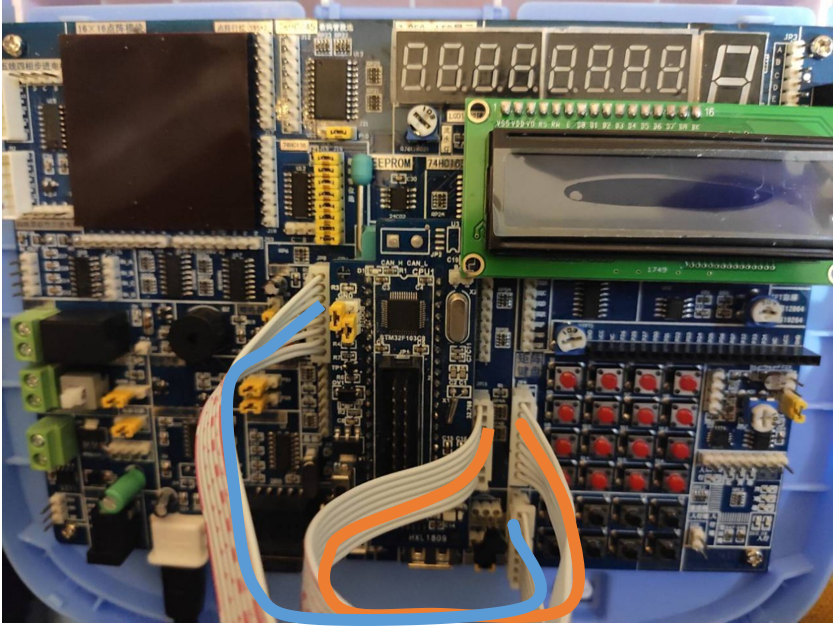2 程序要点
实验利用STM32F10  CPU插件实现扫描键盘数字输入和独立键盘运算符输入以及LCD显示的智能计算器，并实现能够括号运算，加减乘除四则混合运算等较复杂计算的智能计算器。
实验程序主要思路是：使用uvision MDK4编译环境，首先配置系统时钟、NVIC，启动GPIOA、GPIOB模式时钟，设置GPIO I/O口，将LCD1602输出口、矩阵键盘输入口和独立键盘输入口初始化。初始化LCD1602。使用矩阵键盘输入数字，独立键盘输入符号（包括括号等），输入“=”结束输入。如果有输入错误，则清屏，重新输入。输入结束后调用计算器算法calculate()函数，计算输入的表达式结果，再调用result2char()函数将计算结果转换为LCD屏显示的字符，并显示在LCD显示屏上。
2.1 程序流程图3 项目效果
3.1 两位数加法运算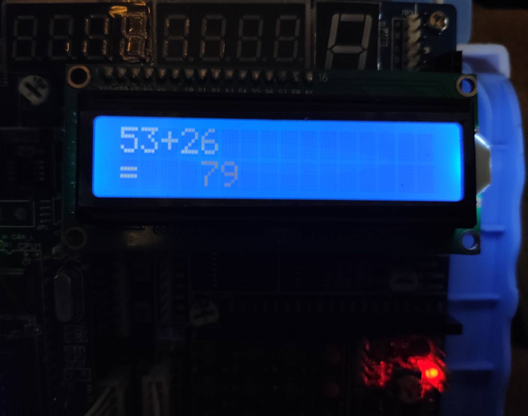3.2 三位数除法运算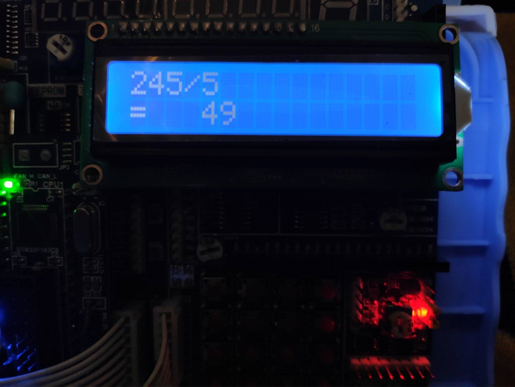3.3 带括号运算的加减乘除四则混合运算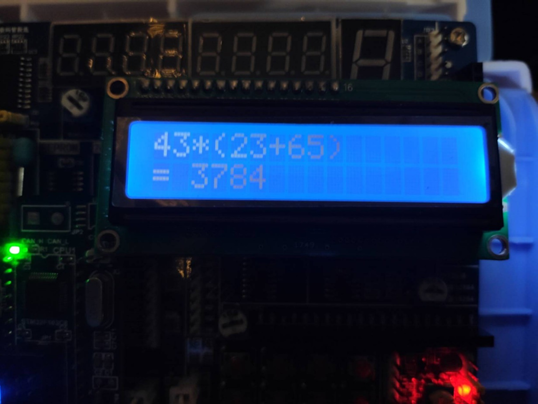4 程序代码
4.0 程序框架和编译环境
使用uvision MDK4编译环境，使用的库函数较少。项目文件框架如下：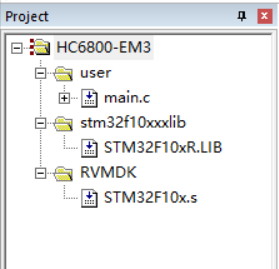只需要把main.c替换成4.3的完整程序代码，再按上图配置lib函数，即可编译成功。
如果配置不便，可以直接下载使用文章首部的 .HEX文件 。将该.HEX文件直接烧录到单片机中即可使用。
4.1 main()主函数
main()
{
j=1;
#ifdef DEBUG
//debug();  //在线调试使用
#endif
RCC_Configuration();      //系统时钟配置函数
NVIC_Configuration();     //NVIC配置函数
RCC_APB2PeriphClockCmd(RCC_APB2Periph_GPIOA | RCC_APB2Periph_GPIOB | RCC_APB1Periph_TIM2 | RCC_APB2Periph_AFIO, ENABLE);//启动GPIO模块时钟
GPIO_PinRemapConfig(GPIO_Remap_SWJ_Disable,ENABLE);  //把调试设置普通IO口
GPIO_InitStructure.GPIO_Pin = GPIO_Pin_All; //所有GPIO为同一类型端口
GPIO_InitStructure.GPIO_Mode = GPIO_Mode_Out_PP;     //推挽输出
GPIO_InitStructure.GPIO_Speed = GPIO_Speed_50MHz;    //输出的最大频率为50HZ
GPIO_Init(GPIOA, &GPIO_InitStructure);   //初始化GPIOA端口
GPIO_Init(GPIOB, &GPIO_InitStructure);   //初始化GPIOB端口
GPIO_InitStructure.GPIO_Pin = GPIO_Pin_8 | GPIO_Pin_9 | GPIO_Pin_10 | GPIO_Pin_11 |GPIO_Pin_12 | GPIO_Pin_13 | GPIO_Pin_14 | GPIO_Pin_15; //所有GPIO为同一类型端口
GPIO_InitStructure.GPIO_Mode = GPIO_Mode_Out_OD;     //开漏输出
GPIO_InitStructure.GPIO_Speed = GPIO_Speed_50MHz;    //输出的最大频率为50HZ
GPIO_Init(GPIOB, &GPIO_InitStructure);   //初始化GPIOB端口
GPIO_Write(GPIOA,0xffff);  //将GPIOA 16个端口全部置为高电平
GPIO_Write(GPIOB,0xffff);  //将GPIOB 16个端口全部置为高电
lcd_init();               // 初始化LCD
delay(10);

while(1)
{
//输入表达式：矩阵键盘输入数字，独立键盘输入符号
while(flag_buf==0 && flag_fuhao2==0 && flag ==0)
{
keydown();     //矩阵按键检测
delay(20);
duli_keyscan();  //独立按键检测
delay(20);
lcd_wcmd_4bit(0x01);//清除LCD的内容
delay(2000);
LCD();     //实时显示按键的表达式
}
//如果有输入数字，将其显示在LCD屏上
if(flag_buf!=0)
{
dis1[j]=dis_buf+48;
}
//如果有输入符号，将其显示在LCD屏上
else if(flag_fuhao2!=0)
{
dis1[j]=fuhao;
}
//清除flag值
j++;
flag_buf=0;
flag_fuhao=0;
flag_fuhao2=0;
if(flag==1)
{
calculate(); //计算表达式的值
result2char();//将计算结果转化为字符串
dis2=61;  //输入“=”
flag_fuhao=0;    //清空flag
flag=0;
}
//如果有清屏指令，则清除屏幕，重新开始输入
if(flag==2)
{
flag=0;
j=1;
for(m=0;m<16;m++)
{
dis1[m]=32;
}
for(m=0;m<16;m++)
{
dis2[m]=32;
}
delay(2000);
LCD();
}
}
}

4.2 主要变量和函数
// 主要变量
typedef unsigned char BYTE;
typedef unsigned char BOOL;
BYTE dis1[] = {"H               "};  //显示字符
BYTE dis2[] = {"                "};
BYTE dis_buf=0;            //显示缓存
BYTE temp;               //中间变量
BYTE key;                //键顺序码
BYTE fuhao=32; //存储符号
BYTE flag=0;  //判断是否结束
BYTE flag_buf=0; //缓存键值
BYTE flag_fuhao=0;//符号的flag
BYTE j,m,n;  //循环计数索引
int i=0;  //循环计数索引
int operand = {0};                //数字数组，初始化
int  top_num = -1;  //数组末尾
BYTE oper = {0};                  //操作符数组，初始化
int top_oper = -1;   //数组首部
BYTE* temp_p;                            // 数组指针
BYTE* str = dis1;//字符串指针
BYTE dest;//字符数组，存储结果

// 主要函数
void RCC_Configuration(void);//RCC初始化
void NVIC_Configuration(void);  //NVIC初始化
void NOP(void);     //延时周期
void Delay(vu32 nCount);   //延时程序
void delay0(BYTE x);      //延时子程序
void keyscan(void);      // 矩阵键盘扫描子程序
void duli_keyscan(void);    //独立按键扫描子程序
void keydown(void);       //判断键盘键是否按下
void calculate(void);         //判断键盘键是否按下
void result2char(void);   //计算表达式的值
void LCD(void); //LCD显示
int insert_operand(int *operand , int * top_num ,BYTE num);//数据压入数据栈
int insert_oper (BYTE * oper , int *top_oper , char ch);//操作符压入符号栈
int compare(BYTE *oper , int *top_oper , BYTE ch);//比较操作服优先级
int deal_date(int *operand ,BYTE *oper ,int *top_num, int *top_oper);//进行数据运算

4.3 完整程序代码
（PS:实验代码有点长，其中有些注释不完整，但编译无错误。可结合以上的程序思路和主要变量、函数注释查看）
#include "stm32f10x_lib.h"
/********************************硬件接口定义*********************************/
#define RS  GPIO_Pin_1 //P2^6;
#define RW  GPIO_Pin_2 //P2^5;
#define EN  GPIO_Pin_0 //P2^7;

/********************************宏定义*********************************/
#define    LCD_RS(x)  x ? GPIO_SetBits(GPIOB, RS): GPIO_ResetBits(GPIOB, RS)
#define    LCD_RW(x)  x ? GPIO_SetBits(GPIOB, RW): GPIO_ResetBits(GPIOB, RW)
#define    LCD_EN(x)  x ? GPIO_SetBits(GPIOB, EN): GPIO_ResetBits(GPIOB, EN)

/********************** 变量定义 ---------------------------*/
GPIO_InitTypeDef GPIO_InitStructure;     //GPIO
ErrorStatus HSEStartUpStatus;
typedef unsigned char BYTE;
typedef unsigned char BOOL;

BYTE dis1[] = {"H               "};
BYTE dis2[] = {"                "};
BYTE dis_buf=0;            //显示缓存
BYTE temp;
BYTE key;                //键顺序码
BYTE fuhao=32;
BYTE flag=0;
BYTE flag_buf=0;
BYTE flag_fuhao=0;
BYTE flag_fuhao2=0;
BYTE j,m,n;
int i=0;
int operand = {0};                /*数字数组，初始化*/
int  top_num = -1;
BYTE oper = {0};                  /*操作符数组，初始化*/
int top_oper = -1;
BYTE* temp_p;							 // 数组指针
BYTE* str = dis1;
BYTE dest;
int num = 0;

/***************声明函数 ---------------*/
void RCC_Configuration(void);//RCC初始化
void NVIC_Configuration(void);	//NVIC初始化
void NOP(void);		//延时周期
void Delay(vu32 nCount);   //延时程序
void delay0(BYTE x);      //延时子程序
void keyscan(void);		 // 矩阵键盘扫描子程序
void duli_keyscan(void); 	//独立按键扫描子程序
void keydown(void);		  //判断键盘键是否按下
void calculate(void);		  //判断键盘键是否按下
void result2char(void);	  //计算表达式的值
void LCD(void); //LCD显示
int insert_operand(int *operand , int * top_num ,BYTE num);//数据压入数据栈
int insert_oper (BYTE * oper , int *top_oper , char ch);//操作符压入符号栈
int compare(BYTE *oper , int *top_oper , BYTE ch);//比较操作服优先级
int deal_date(int *operand ,BYTE *oper ,int *top_num, int *top_oper);//进行数据运算

//RCC初始化
void RCC_Configuration(void)
{
//复位RCC外部设备寄存器到默认值
RCC_DeInit();
//打开外部高速晶振
RCC_HSEConfig(RCC_HSE_ON);
//等待外部高速时钟准备好
HSEStartUpStatus = RCC_WaitForHSEStartUp();
if(HSEStartUpStatus == SUCCESS)   //外部高速时钟已经准别好
{
//开启FLASH的预取功能
FLASH_PrefetchBufferCmd(FLASH_PrefetchBuffer_Enable);
//FLASH延迟2个周期
FLASH_SetLatency(FLASH_Latency_2);
//配置AHB(HCLK)时钟=SYSCLK
RCC_HCLKConfig(RCC_SYSCLK_Div1);
//配置APB2(PCLK2)钟=AHB时钟
RCC_PCLK2Config(RCC_HCLK_Div1);
//配置APB1(PCLK1)钟=AHB 1/2时钟
RCC_PCLK1Config(RCC_HCLK_Div2);
//配置PLL时钟 == 外部高速晶体时钟*9  PLLCLK = 8MHz * 9 = 72 MHz
RCC_PLLConfig(RCC_PLLSource_HSE_Div1, RCC_PLLMul_9);
//使能PLL时钟
RCC_PLLCmd(ENABLE);
//等待PLL时钟就绪
while(RCC_GetFlagStatus(RCC_FLAG_PLLRDY) == RESET)
{
}
//配置系统时钟 = PLL时钟
RCC_SYSCLKConfig(RCC_SYSCLKSource_PLLCLK);
//检查PLL时钟是否作为系统时钟
while(RCC_GetSYSCLKSource() != 0x08)
{
}
}
}

//NVIC初始化
void NVIC_Configuration(void)
{
NVIC_InitTypeDef NVIC_InitStructure;
#ifdef  VECT_TAB_RAM
NVIC_SetVectorTable(NVIC_VectTab_RAM, 0x0);
#else  /* VECT_TAB_FLASH  */
NVIC_SetVectorTable(NVIC_VectTab_FLASH, 0x0);
#endif
NVIC_InitStructure.NVIC_IRQChannel = TIM2_IRQChannel;
NVIC_InitStructure.NVIC_IRQChannelPreemptionPriority = 0;
NVIC_InitStructure.NVIC_IRQChannelSubPriority = 0;
NVIC_InitStructure.NVIC_IRQChannelCmd = ENABLE;
NVIC_Init(&NVIC_InitStructure);
}
#ifdef  DEBUG

//**延时函数*
void NOP()
{ vu16 i;
for(i=0; i<100; i++);
}

//延时函数
void delay(vu32 nCount)
{
for(; nCount != 0; nCount--);
}

void assert_failed(u8* file, u32 line)
{
while (1)
{
}
}
#endif

//延时子程序
void  delay0(BYTE x)
{ BYTE j;
while((x--)!=0)  //CPU执行x*12次
{ for(j=0;j<125;j++)
{;}
}
}

void result2char(void)	  //将计算结果转化为可显示的字符串
{
dis2=operand%10+48;
dis2=operand/10%10+48;
dis2=operand/100%10+48;
dis2=operand/1000%10+48;
dis2=operand/10000%10+48;
dis2=operand/100000%10+48;
if(dis2==48)
{  dis2=32;
if(dis2==48)
{	dis2=32;
if(dis2==48)
{	dis2=32;
if(dis2==48)
{	dis2=32;
if(dis2==48)
{	dis2=32; }
}
}
}
}
}

void calculate(void)		  //判断键盘键是否按下
{
i = 0;
str++;
while(*str != '\0')
{
temp_p = dest;
m=0;
while(*str >= 48 && *str <= 57)           /*判断是否是数据*/
{
*temp_p = *str;
str ++;
temp_p ++;
m++;
}                               /*遇到符号退出*/
if(*str != '(' && *(temp_p - 1) != '\0')      /*判断符号是否为'('*/
{
*temp_p = '\0';
num = atoi(dest);               /*将字符串转为数字*/
insert_operand(operand, &top_num,num);      /*将数据压入数据栈*/
}
while(1)
{
i = compare(oper,&top_oper,*str);      /*判断操作符优先级*/
if(i == 0)
{
insert_oper(oper,&top_oper,*str);   /*压入操作符*/
break;
}
else if(i == 1)                         /*判断括号内的表达式是否结束*/
{
str++;
}
else if(i == -1)                        /*进行数据处理*/
{
deal_date(operand,oper,&top_num,&top_oper);
}
}
str ++;
}
}

//键扫描子程序  (4*3 的矩阵) P0.4 P0.5 P0.6 P0.7为行
void keyscan(void)
{ 	temp = 0;
GPIO_InitStructure.GPIO_Pin = GPIO_Pin_0 |  GPIO_Pin_1 |  GPIO_Pin_2 |  GPIO_Pin_3 ; // 选择所有脚
GPIO_InitStructure.GPIO_Mode = GPIO_Mode_Out_PP;  //配置成推挽式输出
GPIO_InitStructure.GPIO_Speed = GPIO_Speed_50MHz; //输出模式下 I/O输出速度 50M HZ
GPIO_Init(GPIOB, &GPIO_InitStructure);  //初PB口始化
GPIO_InitStructure.GPIO_Pin = GPIO_Pin_4 |  GPIO_Pin_5 |  GPIO_Pin_6 |  GPIO_Pin_7 ; // 选择所有脚
GPIO_InitStructure.GPIO_Mode = GPIO_Mode_IN_FLOATING;  //配置成推挽式输出
GPIO_InitStructure.GPIO_Speed = GPIO_Speed_50MHz; //输出模式下 I/O输出速度 50M HZ
GPIO_Init(GPIOB, &GPIO_InitStructure);  //初PB口始化
GPIO_Write(GPIOB,0X00F0); //初始化端口
delay0(1);				 //延时
temp=~((temp>>4)|0x00F0); //将高四位移到地位处处理
if(temp==1)	  // p0.4 被拉低
{    key=1;	  //第一个按键值
flag_buf=1;
}else if(temp==2)   // p0.5 被拉低
{    key=2;	 //第2个按键值
flag_buf=1;
}else if(temp==4)   // p0.6 被拉低
{    key=3;	  //第3个按键值
flag_buf=1;
}else if(temp==8)   // p0.7 被拉低
{     key=4;	   //第4个按键值
flag_buf=1;
}else	key=16;

GPIO_InitStructure.GPIO_Pin = GPIO_Pin_0 |  GPIO_Pin_1 |  GPIO_Pin_2 |  GPIO_Pin_3 ; // 选择所有脚
GPIO_InitStructure.GPIO_Mode = GPIO_Mode_IN_FLOATING;  //配置成推挽式输出
GPIO_InitStructure.GPIO_Speed = GPIO_Speed_50MHz; //输出模式下 I/O输出速度 50M HZ
GPIO_Init(GPIOB, &GPIO_InitStructure);  //初PB口始化
GPIO_InitStructure.GPIO_Pin = GPIO_Pin_4 |  GPIO_Pin_5 |  GPIO_Pin_6 |  GPIO_Pin_7 ; // 选择所有脚
GPIO_InitStructure.GPIO_Mode = GPIO_Mode_Out_PP;  //配置成推挽式输出
GPIO_InitStructure.GPIO_Speed = GPIO_Speed_50MHz; //输出模式下 I/O输出速度 50M HZ
GPIO_Init(GPIOB, &GPIO_InitStructure);  //初PB口始化
GPIO_Write(GPIOB,0X000F);
delay0(1);			 //延时
temp=~(temp|0xF0);
if(temp==1)		   //p0.0  被拉低
{    key=key+0;
flag_buf=1;
}else if(temp==2)		   //p0.1  被拉低
{    key=key+4;
flag_buf=1;
}else if(temp==4)   //p0.2  被拉低
{    key=key+8;
flag_buf=1;
}else if(temp==8)	//p0.3  被拉低
{   key=key+12;
flag_buf=1;
}else   key=16;

if(key==11) {fuhao=40; flag_fuhao=1	;flag_fuhao2=1;flag_buf=0;}
if(key==12) {fuhao=41; flag_fuhao=1	;flag_fuhao2=1;flag_buf=0;}
dis_buf = key;	    //键值入显示缓存
dis_buf = dis_buf & 0x0f;
}

//独立键盘扫描子程序  (2*4 的矩阵) P0.4 P0.5 P0.6 P0.7为行
void  duli_keyscan(void)
{
temp = 0;
GPIO_InitStructure.GPIO_Pin = GPIO_Pin_All; //所有GPIO为同一类型端口
GPIO_InitStructure.GPIO_Mode = GPIO_Mode_Out_PP;	 //推挽输出
GPIO_InitStructure.GPIO_Speed = GPIO_Speed_50MHz;	 //输出的最大频率为50HZ
GPIO_Init(GPIOA, &GPIO_InitStructure);   //初始化GPIOA端口

GPIO_InitStructure.GPIO_Pin = GPIO_Pin_0 |  GPIO_Pin_1 | GPIO_Pin_2 |  GPIO_Pin_3 | GPIO_Pin_4 |  GPIO_Pin_5 |  GPIO_Pin_6 |  GPIO_Pin_7 ; // 选择所有脚
GPIO_InitStructure.GPIO_Mode = GPIO_Mode_IN_FLOATING;  //配置成推挽式输出
GPIO_InitStructure.GPIO_Speed = GPIO_Speed_50MHz; //输出模式下 I/O输出速度 50M HZ
GPIO_Init(GPIOA, &GPIO_InitStructure);  //初PB口始化

GPIO_Write(GPIOA,0X00FF); //初始化端口
delay0(1);				 //延时
temp=~temp; //将高四位移到地位处处理
if(temp==16)	  // p0.4 被拉低
{   fuhao=43;	  //第一个按键值
flag_fuhao=1;
flag_fuhao2=1;
}
else if(temp==32)   // p0.5 被拉低
{    fuhao=45;	 //第2个按键值
flag_fuhao=2;
flag_fuhao2=1;
}
else if(temp==64)   // p0.6 被拉低
{    fuhao=42;	  //第3个按键值
flag_fuhao=3;
flag_fuhao2=1;
}
else if(temp==128)   // p0.7 被拉低
{    fuhao=47;	   //第4个按键值
flag_fuhao=4;
flag_fuhao2=1;
}
else if(temp==4)   // p0.6 被拉低
{    fuhao=40;	  //第3个按键值
flag_fuhao=3;
flag_fuhao2=1;
}
else if(temp==8)   // p0.7 被拉低
{    fuhao=41;	   //第4个按键值
flag_fuhao=4;
flag_fuhao2=1;
}
else if(temp==1)
{    //fuhao=61;
flag=1;
}
else if(temp!=0)
{	 flag=2;}
}

//函数：判断键是否按下
void  keydown(void)
{   int retval;
//P1=0xF0;       //将高4位全部置1 低四位全部置0
GPIO_InitStructure.GPIO_Pin = GPIO_Pin_0 |  GPIO_Pin_1 |  GPIO_Pin_2 |  GPIO_Pin_3 ; // 选择所有脚
GPIO_InitStructure.GPIO_Mode = GPIO_Mode_Out_PP;  //配置成推挽式输出
GPIO_InitStructure.GPIO_Speed = GPIO_Speed_50MHz; //输出模式下 I/O输出速度 50M HZ
GPIO_Init(GPIOB, &GPIO_InitStructure);  //初PB口始化

GPIO_InitStructure.GPIO_Pin = GPIO_Pin_4 |  GPIO_Pin_5 |  GPIO_Pin_6 |  GPIO_Pin_7 ; // 选择所有脚
GPIO_InitStructure.GPIO_Mode = GPIO_Mode_IN_FLOATING;  //配置成悬浮输入
GPIO_InitStructure.GPIO_Speed = GPIO_Speed_50MHz; //输出模式下 I/O输出速度 50M HZ
GPIO_Init(GPIOB, &GPIO_InitStructure);  //初PB口始化

GPIO_Write(GPIOB,0X00F0);
//P1!=0xF0
if(retval !=0xF0)  //判断按键是否按下 如果按钮按下 会拉低P1其中的一个端口
{
keyscan();  //调用按键扫描程序
}
}

/*数据压入数据栈*/
int insert_operand(int *operand , int * top_num ,BYTE num)           /*数据压入数据栈*/
{
(*top_num) ++;
operand[*top_num] = num;                    /*保存数据*/
return 0;                           /*正常退出*/
}

/*操作符压入符号栈*/
int insert_oper (BYTE * oper , int *top_oper , char ch)             /*操作符压入符号栈*/
{
(*top_oper)++;
oper[*top_oper] = ch;                       /*保存操作符*/
return 0;                           /*正常退出*/
}

/*比较操作服优先级*/
int compare(BYTE *oper , int *top_oper , BYTE ch)                   /*比较操作服优先级*/
{
if((oper[*top_oper] == '-' || oper[*top_oper] == '+')           /*判断当前优先级是否比栈顶操作符优先级高*/
&& (ch == '*' || ch == '/'))
{
return 0;                      /*操作符压入栈*/
}
else if(*top_oper == -1 || ch == '('
|| (oper[*top_oper] == '(' && ch != ')'))       /*判断操作符栈是否为空；栈顶操作                                                               符是否为'('*/
{
return 0;                       /*操作符压入栈*/
}
else if (oper[*top_oper] =='(' && ch == ')' )       /*判断括号内的表达式是否计算完毕*/
{
(*top_oper)--;
return 1;                       /*对（）进行处理*/
}
else
{
return -1;                                          /*进行操作符的运算*/
}
}

/*进行数据运算*/
int deal_date(int *operand ,BYTE *oper ,int *top_num, int *top_oper)    /*进行数据运算*/
{
int num_1 = operand[*top_num];              /*取出数据栈中两个数据*/
int num_2 = operand[*top_num - 1];
int value = 0;
if(oper[*top_oper] == '+')                  /*加法操作*/
{
value = num_1 + num_2;
}
else if(oper[*top_oper] == '-')             /*减法操作*/
{
value = num_2 - num_1;
}
else if(oper[*top_oper] == '*')             /*乘法操作*/
{
value = num_2 * num_1;
}
else if(oper[*top_oper] == '/')             /*除法操作*/
{
value = num_2 / num_1;
}
(*top_num) --;                              /*将数据栈顶下移一位*/
operand[*top_num] = value;                  /*将得到的值压入数据栈*/
(*top_oper) --;                             /*将操作符栈顶下移一位*/
}

/***********测试LCD繁忙状态**********************/
BOOL lcd_bz()
{                         // 测试LCD忙碌状态
BOOL result;
LCD_RS(0);  //0;
LCD_RW(1);  //1;
LCD_EN(1);  //1;
NOP();
NOP();
LCD_EN(0); // 0;
return result;
}
/*********写命令****************/
lcd_wcmd(long cmd)
{                          // 写入指令数据到LCD
//	while(lcd_bz());
LCD_RS(0); // 0;
LCD_RW(0); // 0;
LCD_EN(0); // 0;
NOP();
NOP();
GPIOB->BSRR = cmd<<8 & 0xf000;  //将数据送到P0口
GPIOB->BRR = ((~cmd)<<8) & 0xf000;
NOP();
NOP();
LCD_EN(1); // 1;
NOP();
NOP();
LCD_EN(0); // 0;
}

lcd_wcmd_4bit(long cmd)
{                          // 写入指令数据到LCD
while(lcd_bz());
LCD_RS(0); // 0;
LCD_RW(0); // 0;
LCD_EN(0); // 0;
NOP();
NOP(); 	 NOP();
NOP();
GPIOB->BSRR = cmd<<8 & 0xf000;  //将数据送到P0口
GPIOB->BRR = ((~cmd)<<8) & 0xf000;
NOP();	 NOP();
NOP();
NOP();
LCD_EN(1); // 1;
NOP();
NOP();	NOP();
NOP();
LCD_EN(0); // 0;
LCD_RS(0); //= 1;
LCD_RW(0); //= 0;
LCD_EN(0); //= 0;
NOP();
NOP(); 	 NOP();
NOP();
GPIOB->BSRR = cmd<<12 & 0xf000;  //将数据送到P0口
GPIOB->BRR = ((~cmd)<<12) & 0xf000;
NOP();
NOP();	 NOP();
NOP();
LCD_EN(1); // 1;
NOP();
NOP();	NOP();
NOP();
LCD_EN(0); // 0;
}

lcd_pos(long pos)
{                          //设定显示位置
lcd_wcmd_4bit(pos | 0x0080);
}

lcd_init()				//LCD初始化设定
{
lcd_wcmd(0x38);          //16*2显示，5*7点阵，8位数据
delay(20000);
lcd_wcmd(0x38);          //16*2显示，5*7点阵，8位数据										/
delay(20000);
lcd_wcmd(0x28);          //16*2显示，5*7点阵，8位数据
delay(20000);
lcd_wcmd_4bit(0x28);          //16*2显示，5*7点阵，8位数据
delay(20000);
lcd_wcmd_4bit(0x0c);          //显示开，关光标
delay(20000);
lcd_wcmd_4bit(0x02);          //移动光标
delay(20000);
lcd_wcmd_4bit(0x01);          //清除LCD的显示内容
delay(20000);
}

/*****************写数据***********************/
lcd_wdat( long dat)
{                          //写入字符显示数据到LCD
while(lcd_bz());
LCD_RS(1); //= 1;
LCD_RW(0); //= 0;
LCD_EN(0); //= 0;

GPIOB->BSRR = dat<<8 & 0xf000;    ////P0 = dat
GPIOB->BRR = ((~dat)<<8) & 0xf000;
NOP();
NOP();
NOP();
NOP();
LCD_EN(1);// = 1;
NOP();
NOP();
NOP();
NOP();
LCD_EN(0);// = 0;

LCD_RS(1); //= 1;
LCD_RW(0); //= 0;
LCD_EN(0); //= 0;
NOP();
NOP();
NOP();
NOP();
GPIOB->BSRR = dat<<12 & 0xf000;    ////P0 = dat
GPIOB->BRR = ((~dat)<<12) & 0xf000;
NOP();
NOP();
NOP();
NOP();
LCD_EN(1);// = 1;
NOP();
NOP();
NOP();
NOP();
LCD_EN(0);// = 0;
}

void LCD(void)
{
lcd_wcmd_4bit(0x06);            //向右移动光标
lcd_pos(0);                //设置显示位置为第一行的第1个字符
i = 0;
while(dis1[ i ] != '\0')
{                           //显示字符
lcd_wdat(dis1[ i ]);
i++;
delay(20000);                //控制两字之间显示速度
}
lcd_pos(0x40);             //设置显示位置为第二行第1个字符
i = 0;
while(dis2[ i ] != '\0')
{
lcd_wdat(dis2[ i ]);      //显示字符
i++;
delay(20000);                //控制两字之间显示速度
}
delay(800000);                //控制停留时间
}

main()
{
j=1;
#ifdef DEBUG
//debug();  //在线调试使用
#endif
RCC_Configuration();      //系统时钟配置函数
NVIC_Configuration();     //NVIC配置函数
RCC_APB2PeriphClockCmd(RCC_APB2Periph_GPIOA | RCC_APB2Periph_GPIOB | RCC_APB1Periph_TIM2 | RCC_APB2Periph_AFIO, ENABLE);//启动GPIO模块时钟
GPIO_PinRemapConfig(GPIO_Remap_SWJ_Disable,ENABLE);  //把调试设置普通IO口
GPIO_InitStructure.GPIO_Pin = GPIO_Pin_All; //所有GPIO为同一类型端口
GPIO_InitStructure.GPIO_Mode = GPIO_Mode_Out_PP;	 //推挽输出
GPIO_InitStructure.GPIO_Speed = GPIO_Speed_50MHz;	 //输出的最大频率为50HZ
GPIO_Init(GPIOA, &GPIO_InitStructure);   //初始化GPIOA端口
GPIO_Init(GPIOB, &GPIO_InitStructure);   //初始化GPIOB端口
GPIO_InitStructure.GPIO_Pin = GPIO_Pin_8 | GPIO_Pin_9 | GPIO_Pin_10 | GPIO_Pin_11 |GPIO_Pin_12 | GPIO_Pin_13 | GPIO_Pin_14 | GPIO_Pin_15; //所有GPIO为同一类型端口
GPIO_InitStructure.GPIO_Mode = GPIO_Mode_Out_OD;	 //开漏输出
GPIO_InitStructure.GPIO_Speed = GPIO_Speed_50MHz;	 //输出的最大频率为50HZ
GPIO_Init(GPIOB, &GPIO_InitStructure);   //初始化GPIOB端口
GPIO_Write(GPIOA,0xffff);  //将GPIOA 16个端口全部置为高电平
GPIO_Write(GPIOB,0xffff);  //将GPIOB 16个端口全部置为高电
lcd_init();               // 初始化LCD
delay(10);

while(1)
{
//输入表达式：矩阵键盘输入数字，独立键盘输入符号
while(flag_buf==0 && flag_fuhao2==0 && flag ==0)
{
keydown();	 //矩阵按键检测
delay(20);
duli_keyscan();  //独立按键检测
delay(20);
lcd_wcmd_4bit(0x01);//清除LCD的内容
delay(2000);
LCD();	 //实时显示按键的表达式
}
//如果有输入数字，将其显示在LCD屏上
if(flag_buf!=0)
{
dis1[j]=dis_buf+48;
}
//如果有输入符号，将其显示在LCD屏上
else if(flag_fuhao2!=0)
{
dis1[j]=fuhao;
}
//清除flag值
j++;
flag_buf=0;
flag_fuhao=0;
flag_fuhao2=0;
if(flag==1)
{
calculate(); //计算表达式的值
result2char();//将计算结果转化为字符串
dis2=61;  //输入“=”
flag_fuhao=0;	  //清空flag
flag=0;
}
//如果有清屏指令，则清除屏幕，重新开始输入
if(flag==2)
{
flag=0;
j=1;
for(m=0;m<16;m++)
{
dis1[m]=32;
}
for(m=0;m<16;m++)
{
dis2[m]=32;
}
delay(2000);
LCD();
}
}
}


程序可能还有一些不完善的地方，欢迎交流！


展开全文单片机 objective-c 经验分享
• 基于51单片机的数码管和矩阵按键实现的简易计算器，其实可以加入的东西有很多，就像我这个负数运算还没有加进去，以及我的除法运算只能算655（65535/100）以内的数，不过就先做到这里，这个作品至少已经叫做计算器了...
基于51单片机的数码管和矩阵按键实现的简易计算器，其实可以加入的东西有很多，就像我这个负数运算还没有加进去，以及我的除法运算只能算655（65535/100）以内的数，不过就先做到这里，这个作品至少已经叫做计算器了。
首先我这个工程里加入了两个文件，数码管显示文件，查询式矩阵按键文件，直接调用这两块功能的函数，然后在主函数里解决相当于软件层的计算器功能。
简易计算器实现的功能（待完善）

四则运算
可以显示2位小数
利用矩阵按键输入，用数码管显示
连续运算
负数运算

代码的解释和功能实现
其它函数说明：
/*定义局部函数*/
void deletenum(int s[]);//退位函数，用于退1位，等同'c'
void count(void);//计算函数等同'='
unsigned long pow10(u8 n);//计算10的n次方
void init(void);//计算完成后初始化第二个输入数
void division(void);//除法运算
void multiplication(void);//乘法运算
void subtraction(void);//减法运算


/*定义变量*/
int gDigValue;//gDigValue用于显示数码管作为全局变量
int val,a,b;//分别存放运算结果，第一个数，第二个数
unsigned long sum=0,um=0;//sum用于存储被除数
u8 num=0,ch=0;//ch存放运算符，num存放键值
u8 flag1=0,flag2=1,next_flag=1;//分别代表是否有小数点，是否显示运算结果，是否要输入下一个数

/*主函数
用于放置主要流程：1.获取键值，根据键值进行操作 2.分情况将数值显示在数码管
（输入数字时显示正在输入的数，运算后显示结果）
*/
void main(void)
{
while(1)
{
num=key_val();//获取键值
if(num!=17)
flag2=1;//当有按键按下，不再显示运算结果，改为显示正在输入的数
WaitForKeyUp();
if(next_flag)
{
if(num>9)
{
switch(num)
{
case 14:deletenum(a);break;//退位
case 15:break;//没有输入运算符的时候不能计算
case 17:break;//没有按键按下
default:ch=num;next_flag=0;break;//当按下的是运算符，用ch先保存，然后输入下一个数的标志next_flag等于0(逻辑否)
}
}
else
{
a=num;
}
}
else
{
if(num>9)
{
switch(num)
{
case 14:deletenum(b);break;
case 15:count();break;//在输入运算符后可以计算
case 17:break;//没有按键按下
default:ch=num;break;
}
}
else//如果输入数字，所有数字左移一格，然后输入新的数字
{
b=num;
}
}
if(flag2)//是否显示运算结果
{
if(next_flag)//显示正在输入的数
for(u8 i=0;i<8;i++)
{
gDigValue[i]=a[i];
}
else
for(u8 i=0;i<8;i++)
{
gDigValue[i]=b[i];
}
}
else
{
if(flag1 && val==0 && val==0)//如果没有小数位(小数点后两位为0),则去掉小数点，所有数字右移两格
{
flag1=0;//不再显示小数点
for(int j=7;j>=2;j--)//右移两格
{
val[j]=val[j-2];
}
val=0;
val=0;
}
for(u8 i=0;i<8;i++)
{
a[i]=val[i];//让第一个数变为运算的结果，可以连续运算
gDigValue[i]=val[i];//显示运算结果
}
}
DigDisplayVal();//数码管显示数值
}

}


/*用于获取键值，方便键值的改变，包含在key.c文件中*/
u8 key_val(void)
{
switch(KeyScan())//获得查询式获取键值的结果
{
case 1:return 7;break;//数字7
case 2:return 8;break;//数字8
case 3:return 9;break;//数字9
case 4:return 11;break;// / 除号
case 5:return 4;break;//数字4
case 6:return 5;break;//数字5
case 7:return 6;break;//数字6
case 8:return 12;break;// * 乘号
case 9:return 1;break;//数字1
case 10:return 2;break;//数字2
case 11:return 3;break;//数字3
case 12:return 13;break;// - 减号
case 13:return 14;break;// 退位
case 14:return 0;break;//数字0
case 15:return 15;break;// = 等于
case 16:return 16;break;// + 加号
default:return 17;//没有按下按键时返回17
}
}

四则运算函数，除了除法
，其他的类比列式计算
是不是生动形象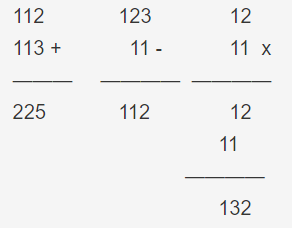/*减法运算*/
void subtraction(void)
{
for(int i=7;i>=0;i--)
{
val[i]=a[i]-b[i];
if(val[i]<0)//小于0的要借位
{
val[i]+=10;
a[i-1]-=1;
}
}
}

/*加法运算*/
{
for(int i=7;i>=0;i--)
{
val[i]=a[i]+b[i];
if(val[i]>9)//大于10的要进位
{
val[i]-=10;
val[i-1]+=1;
}
}
}
/*乘法运算*/
void multiplication(void)
{
for(int i=7;i>=0;i--)//类比我们的列式乘法，将乘数的每一位分别和被乘数的每一位相乘。
{
for(int j=7;j>=0;j--)
{
if(i+j>=7)
val[i-7+j]+=a[i]*b[j];
}
}
for(int i=7;i>=0;i--)//将每一位大于10的进位
while(val[i]>9)
{
val[i]-=10;
val[i-1]+=1;
}
}
/*除法运算，这个算法受51单
片机运算能力限制只能运算输
入数字在655以内的除法。顺
带一提，要显示小数点，我们
需要回到数码管显示的底层函
数里，将段码|(位与)一个0x80，
也就是DIG_PORT=gDuanMa[gDigVal[i]] | 0x80;
*/
void division(void)
{
for(int i=7;i>=0;i--)
{
sum=sum+a[i]*pow10(7-i);//将输入的第一个数(被除数)获取
um=um+b[i]*pow10(7-i);//将输入的第二个数(除数)获取
}
if(sum<1000000)//共两位小数点，所以整数部分最多6位，实际上到不了那么多位
{
sum=(sum*100)/um;
for(int i=0;i<8;i++)
{
val[7-i]=(sum%pow10(i+1))/pow10(i);
}
flag1=1;
}
}




展开全文51单片机
• 教程及网络上大部分只有单一简单的4则运算的例子,这里写一个可以连续运算功能的,清零则重新开始.代码如下: 可在=运算后还能连续运算，除非清零从新开始，都可以连续运算，如：1+2+3+4+5+6+7+8+9=45-5=40+1+2+10=...
• 今天，我在Keil uVision4中制作了一个小项目，利用STC29C52单片机实现了简易计算器的功能。 此计算器利用矩阵键盘（线扫描法）输入数据，利用八位数码管显示数据。能实现四位整数（及四位以下）与四位整数（及四位...单片机
• at89c51单片机 计算器 矩阵键盘 c语言代码 课程设计
• 如何利用廉价的51单片机来控制网卡芯片进行数据传输，加载TCP/IP协议连接到互联网，实现网络通信成了众多设计者的目标。但由于指令及资源的限制，实施过程会有许多困难。我们在设计方案中舍弃了耗费资源的高级协议，...51单片机
• 电子设计论文单片机实现各种控制策略和算法的载体。由台湾凌阳公司生产的SPCE系列单片机，因其功耗低，超小型，低成本，功能完整，非常适用于便携式仪表和就地式显示控制仪表，在国内越来越受到用户的重视和广泛的...编程 算法 产品设计 存储系统
• 基于STC89C51单片机的温湿度检测系统 摘 要：设计一种计算机教室温度、湿度检测系统，用于控制机房的温、湿度。整个系统采用STC89C51微处理器作为主控系统，硬件电路主要包括51单片机、DHT11温湿度传感器、显示器...51单片机
• 流水灯模块接在单片机的P1口，由原理图可以知道，在P1口给一个低电平即可点亮LED灯。相反，如果要LED灯熄灭，就要把P1口的电平变为高电平即可。要实现流水灯功能，我们只要将LED1～LED8依次点亮、熄灭，依始类推，8...
• 1 TITLE MASM Template (main.asm) 2 3 ; Description: 4 ; 5 ; Revision date: 6 7 .386P ; Pentium Pro or later 8 .MODEL flat, stdcall 9 .STACK 4096 10 option casemap:none; ...
• 题目要求：进行有理数的四则运算重载 实现如下这样的运算：  1/8 + 7/8 = 1;  1/8 - 7/8 = -3/4;  1/8 * 7/8 = 7/64;  1/8 / 7/8 = 1/7; 说明：这其实考察的就是C++中运算符的重载问题，这个时候因为没有...运算符重载
• 这里使用的是AT89S51，利用定时器方式1实现跑马灯可以以5种速度变换（速度可以增加，只需要修改一点点的代码），并实现4种花样（用K1-K4键进行控制），也使用到了点阵。 实现过程 由于之前有做过按下按键使数码管...单片机
• 单片机基础-第一个单片机系统 简单的单片机系统 ...如果把单片机和外围器件组合起来，实现一定的功能，那我们就称单片机和外围器件组成了单片机系统。 如何控制一个发光二极管——闪烁 500ms ...单片机
...